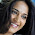## Lets Learn Pandas

In :
```import pandas as pd
```

### Pandas series

pandas series is similar to numpy array, But it support lots of extra functionality like Pandaseries.describe()
Basic access is similar to numpy array, it support access by index( s ) or slicing ( s[5:10] ).
It also support vectorise operation and looping like numpy array.
Implemented in C so it works very fast.

Learn Basic of Numpy

### Benfits of Pandas series¶

In :
```s=pd.Series([2,3,4,5,6])
print s.describe()
```
```count    5.000000
mean     4.000000
std      1.581139
min      2.000000
25%      3.000000
50%      4.000000
75%      5.000000
max      6.000000
dtype: float64
```

### Pandas Index

Hybrid of list and python Dictionary. It map key value pair.
In :
```sal=pd.Series([40,12,43,56],
index=['Ram',
'Syam',
"Rahul",
"Ganesh"])
print sal
```
```Ram       40
Syam      12
Rahul     43
Ganesh    56
dtype: int64
```
In :
```print sal
```
```40
```

#### lookUp by index

In :
```print sal.loc["Syam"]
```
```12
```
Using sal[position] is not prefered instead prefer to use sal.iloc[position] becouse Index has different meaning in series so it avoid confusion
In :
```print sal.iloc
```
```56
```
argmax() function return index of max value element
In :
```print sal.argmax()
```
```Ganesh
```
In :
```print sal.loc["Ganesh"]
print sal.max()
```
```56
56
```

### Adding series with Different index¶

In :
```a=pd.Series([1,2,3,4],
index=["a","b","c","d"])
b=pd.Series([9,8,7,6],
index=["c","d","e","f"])
print a
```
```a    1
b    2
c    3
d    4
dtype: int64
```
In :
```print b
```
```c    9
d    8
e    7
f    6
dtype: int64
```
In :
```print a+b
```
```a   NaN
b   NaN
c    12
d    12
e   NaN
f   NaN
dtype: float64
```

C,D are common in both so added correctly rest are just assign a value NaN (Not a number)

#### we can modify it such that in case of mismatch original data will assign instead of NaN or drop All NaN

In :
```res = (a+b)
print res.dropna()
```
```c    12
d    12
dtype: float64
```

#### Treat missing values as 0¶

In :
```res=a.add(b,fill_value=0)
print res
```
```a     1
b     2
c    12
d    12
e     7
f     6
dtype: float64
```
s.apply(function_name) used to apply some operation on each element.

### Example:

adding 5 to each element , we can do this by simply series+5 because it is a vector, But lets do using this new technique s.apply(function)
In :
```print res
```
```a     1
b     2
c    12
d    12
e     7
f     6
dtype: float64
```
In :
```print res+5
```
```a     6
b     7
c    17
d    17
e    12
f    11
dtype: float64
```
In :
```def add_5(x):
return x+5
```
In :
```print res.apply(add_5)
```
```a     6
b     7
c    17
d    17
e    12
f    11
dtype: float64
```

### Plotting

Automatically plot index vs data plot
In :
```%pylab inline
res.plot()
```
```Populating the interactive namespace from numpy and matplotlib
```
Out:
`<matplotlib.axes.AxesSubplot at 0x5746350>`

#### 1 comment:

1.This comment has been removed by the author.

THANKS FOR UR GREAT COMMENT

Blogger Widgets